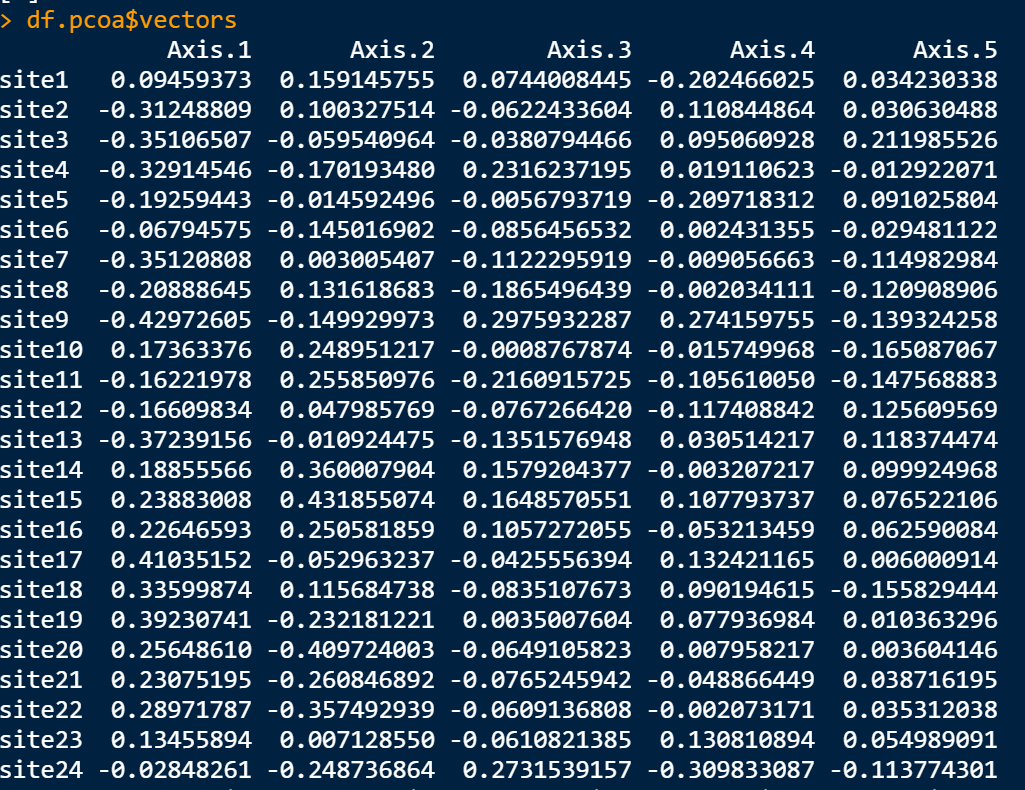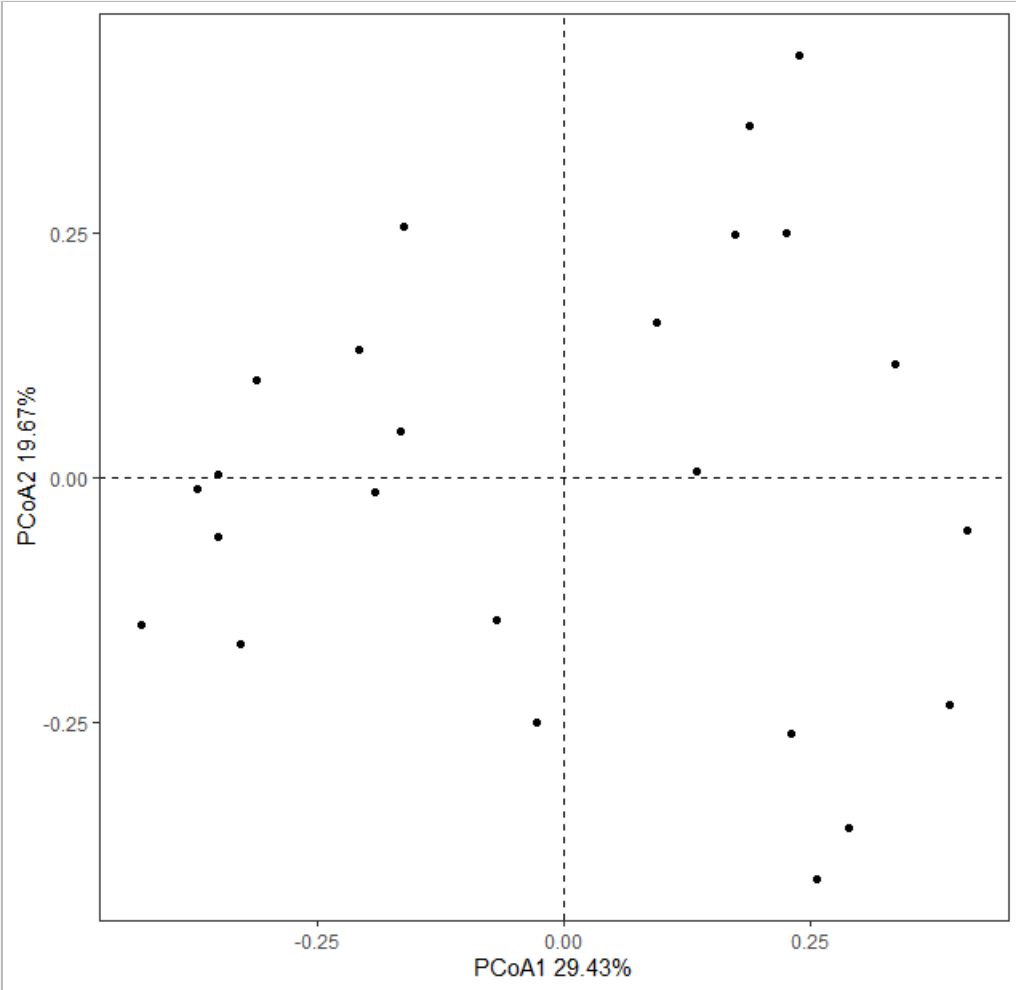R语言做主坐标分析（PCoA）的一个简单小例子

2021/03/31 18:59

library(vegan)

data("varespec")

下面开始实际操作
• 首先是将数据集赋值给新的变量，并以site1-24对新的数据集的行进行命名

df<-varespec
rownames(df)<-paste0("site",1:24)
• 计算距离
bray_dist<-vegdist(df,method = "bray")
• 使用ape这个包中的pcoa()函数做PCoA分析
library(ape)
df.pcoa<-pcoa(bray_dist,correction = "cailliez")

df.pcoa$vectors能够获得用于画图的数据image.png df.pcoa$values可以获得坐标轴上显示的百分比

最后用ggplot2来画这个图
df.plot<-data.frame(df.pcoa$vectors) head(df.plot) library(ggplot2) x_label<-round(df.pcoa$values$Rel_corr_eig*100,2) y_label<-round(df.pcoa$values$Rel_corr_eig*100,2) x_label y_label ggplot(data=df.plot,aes(x=Axis.1,y=Axis.2))+ geom_point()+ theme_bw()+ theme(panel.grid = element_blank())+ geom_vline(xintercept = 0,lty="dashed")+ geom_hline(yintercept = 0,lty="dashed")+ labs(x=paste0("PCoA1 ",x_label,"%"), y=paste0("PCoA2 ",y_label,"%"))image.png 通过上图我们可以看到这些样地大体上可以分为两组，如果自己手头有样地的分组数据就可以看看这个结果是不是和自己的分组数据一致。 下面人为的给他分个组，然后添加一个表示分组的椭圆 df.plot$group<-ifelse(df.plot\$Axis.1<0,"AAA","BBB")
ggplot(data=df.plot,aes(x=Axis.1,y=Axis.2,
color=group,shape=group))+
geom_point(size=5)+
theme_bw()+
theme(panel.grid = element_blank())+
geom_vline(xintercept = 0,lty="dashed")+
geom_hline(yintercept = 0,lty="dashed")+
labs(x=paste0("PCoA1 ",x_label,"%"),
y=paste0("PCoA2 ",y_label,"%"))+
stat_ellipse(data=df.plot,
geom = "polygon",
aes(fill=group),
alpha=0.3)+
scale_fill_manual(values = c("#e31a1c","#1f78b4"))

0
0 收藏

作者的其它热门文章0 评论
0 收藏
0